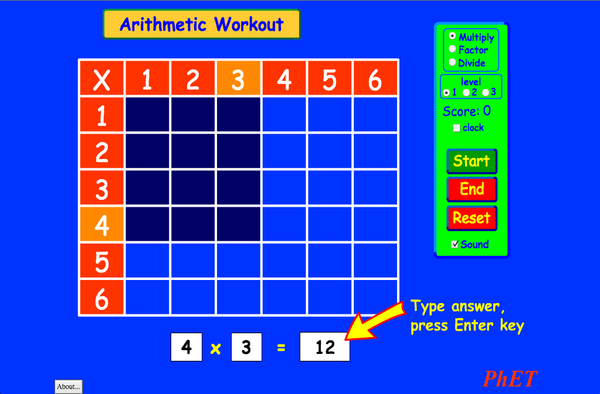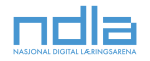AritmetikaStiahnuťKód na vloženie zatvoriť Vložiť bežiacu kópiu tejto simulácie Použite tento HTML kód pre vloženie tejto simulácie na svoju web stránku. Môžete taktiež zmeniť výšku a šírku vloženej simulácie zmenením atribútov "width" a "height" v HTML kóde. Vložiť obrázok, ktorý po kliknutí spustí simuláciu.
Kliknite pre spustenie
Použite tento HTML kód pre zobrazenie obrazovky simulácie s nápisom "Kliknite pre spustenie" Násobenie PhET podporujea pedagógovia ako ste Vy.Späť na HTML5 verziu

• Násobenie

Popis

Pamätáš si dobre násobilku? Precvič si svoje zručnosti násobenia, delenia a rozkladu na súčinitele v tejto vzrušujúcej hre. Kalkulačky nie sú povolené!

Ukážkové ciele vzdelávania

• Objasniť, ako tabuľka násobilky pomáha pri násobení, rozklade na súčinitele a delení.
• Použitím modelu polia pochopiť násobenie, rozklad a delenie.
• Znížiť chybovosť pri násobení, rozklade a delení.
• Vyvinúť rôzne stratégie pre riešenie aritmetických úloh.

Standards Alignment

Common Core - Math

3.OA.A.1
Interpret products of whole numbers, e.g., interpret 5 × 7 as the total number of objects in 5 groups of 7 objects each. For example, describe a context in which a total number of objects can be expressed as 5 × 7.
3.OA.A.2
Interpret whole-number quotients of whole numbers, e.g., interpret 56 ÷ 8 as the number of objects in each share when 56 objects are partitioned equally into 8 shares, or as a number of shares when 56 objects are partitioned into equal shares of 8 objects each. For example, describe a context in which a number of shares or a number of groups can be expressed as 56 ÷ 8.
3.OA.A.4
Determine the unknown whole number in a multiplication or division equation relating three whole numbers. For example, determine the unknown number that makes the equation true in each of the equations 8 × ? = 48, 5 = _ ÷ 3, 6 × 6 = ?
3.OA.B.5
Apply properties of operations as strategies to multiply and divide.2 Examples: If 6 × 4 = 24 is known, then 4 × 6 = 24 is also known. (Commutative property of multiplication.) 3 × 5 × 2 can be found by 3 × 5 = 15, then 15 × 2 = 30, or by 5 × 2 = 10, then 3 × 10 = 30. (Associative property of multiplication.) Knowing that 8 × 5 = 40 and 8 × 2 = 16, one can find 8 × 7 as 8 × (5 + 2) = (8 × 5) + (8 × 2) = 40 + 16 = 56. (Distributive property.)
3.OA.B.6
Understand division as an unknown-factor problem. For example, find 32 ÷ 8 by finding the number that makes 32 when multiplied by 8.
3.OA.C.7
Fluently multiply and divide within 100, using strategies such as the relationship between multiplication and division (e.g., knowing that 8 × 5 = 40, one knows 40 ÷ 5 = 8) or properties of operations. By the end of Grade 3, know from memory all products of two one-digit numbers.
3.OA.D.9
Identify arithmetic patterns (including patterns in the addition table or multiplication table), and explain them using properties of operations. For example, observe that 4 times a number is always even, and explain why 4 times a number can be decomposed into two equal addends.
Verzia 2.03

Typy pre učiteľovPrehľad ovládacích prvkov simulácie, modelové zjednodušenie a postrehy o študentskom uvažovaní. ( PDF ).

Teacher-Submitted Activities

Arithmetic Games (Inquiry Based)Trish Loeblein Lab Matematika
Juego de Aritmética (Basado en Indagación)Trish Loeblein, traducido por Diana López
ZŠ-II

Guided
Matematika
How do PhET simulations fit in my middle school program?Sarah Borenstein ZŠ-II Iné Chémia
Veda o Zemi
Biológia
Fyzika
MS and HS TEK to Sim Alignment Elyse Zimmer
ZŠ-II
Iné Fyzika
Biológia
Chémia
Math facts - Using the Multiplication Chart Tapan Sarkar ZŠ-II Lab Matematika
Back to the Basics Nyetta Abernathy K-5
ZŠ-II
Lab
Matematika
Discovering divisibility rules Stacy Larson ZŠ-II
Lab Matematika
곱셈 나눗셈 연습 SIM 사용설명서 이화국(Wha Kuk Lee) ZŠ-II
K-5

Otaz
Demo
Matematika
Fyzika
OBJETO DE APRENDIZAGEM: ARITHMETIC Arlete Moura de Oliveira Cabral e Maria Cleide da Silva Barroso K-5 Guided Matematika
Operações Aritméticas Fundamentais Georgyana Cidrão; Francisco Régis Vieira Alves K-5 Guided
Lab
Matematika
Jazyk Stiahnuť alebo spustiť Tips
Afrikánčina All AfrikaansRekenkunde
Albánčina All shqipAritmetika
Angličtina All EnglishArithmetic
Arabčina All العربيةarithmetic
Arabčina (Saudská Arábia) All العربية (السعودية)arithmetic
Baskičtina All EuskaraAritmetika
Bosnian All BosanskiARITMETIKA
Čínština (Tradičná) All 中文 (台灣)算數
Čínština (Zjednodušená) All 中文 (中国)算术
Dánčina All DanskAritmetisk
Estónčina All EestiAritmeetika
Francúzština All françaisArithmétique
Gréčtina All ΕλληνικάΑριθμητικός
Gruzínčina All ქართულიარითმეტიკა
Hebrejčina All עבריתחשבון
Holandčina All NederlandsRekenen
Chorvátčina All hrvatskiAritmetika
Indonesian All Bahasa IndonesiaAritmatika
Japončina All 日本語計算
Kazakh All KazakhАрифметика
Kórejčina All 한국어곱셈 나눗셈 연습
Latvian All LatviešuAritmētika
Macedonian All македонскиАРИТМЕТИКА
Maďarčina All magyarSzorzótábla játék
Marathi All मराठीअंकगणित
Nemčina All DeutschRechnen
Perzština All فارسیحساب
Poľština All polskiArytmetyka
Portugalčina (Brazília) All português (Brasil)Aritmética
Rumunčina All românăAritmetica
Ruština All русскийАрифметика
Srbčina All СрпскиАРИТМЕТИКА
Swahili All SwahiliHesabu
Španielčina All españolAritmética
Španielčina (Mexico) All español (México)Aritmética
Španielčina (Peru) All español (Perú)Aritmética
Švédčina All svenskaMatteräkning
Taliančina All italianotabelline
Turečtina All TürkçeAritmetik
Turkmen All TurkmenArifmetika
Vietnamčina All Tiếng ViệtSố học
Windows Macintosh Linux
Microsoft Windows
XP/Vista/7/8.1/10
Macromedia Flash 9 or later
OS X 10.9.5 or later
Macromedia Flash 9 or later
Macromedia Flash 9 or later
Autorský tím Iné knižnice Poďakovanie
• Michael Dubson (developer/lead)
• Trish Loeblein